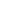# How To Calculate Your Kua Number

The kua number is an important component of practicing 8 mansions feng shui.

It clearly states good and bad areas based on cardinal and intermediate directions.

Once an individual accurately calculates his or her kua number, the 4 favorable and 4 unfavorable directions can be identified.

This will help a homeowner figure the basics of which sections in a house should be kept active or inactive.

The methods for determining kua number is different for men and women.

Calculating Kua number for women

Using the birth year, add up the single digits of the first two numbers and the last two numbers. The add them up using the same methodology until you end up with a single digit. Then add that to 4.

For example, a female born in the year 1978 would be:

(1+9) + (7+8) = 10 + 15 = 25

2 + 5 = 7

7 + 4 = 11

Since the result is 11, the Kua number = 1 + 1 = 2

If the resulting number is 5, the kua number would become 8 by default since the kua number 5 does not exist.

Calculating Kua number of men

Using the birth year, add up the single digits of the first two numbers and the last two numbers. The add them up using the same methodology until you end up with a single digit. The subtract that from 11.

For example if a male is born in the year 1976, the kua number would be:

(1+9) + (7+6) = 10 + 13 = 23

2 + 3 = 5

Kua number = 11 – 5 = 6

If the resulting number is 5, the kua number would become 2 by default since the kua number 5 does not exist.

Good directions according to 8 mansions

The 4 good directions according to 8 mansions feng shui are:

1. Sheng chi (SC)
2. Tien yi (TY)
3. Yen nian (YN)
4. Fu Wei (FW)
 Kua SC TY YN FW 1 SE E S N 2 NE W NW SW 3 S N SE E 4 N S E SE 6 W NE SW NW 7 NW SW NE W 8 SW NW W NE 9 E SE N S

Bad directions according to 8 mansions

The 4 good directions according to 8 mansions feng shui are:

1. Jue Ming (JM)
2. Liu Sha (LS)
3. Wu Gui (WG)
4. Huo Hai (HH)
 Kua JM LS WG HH 1 SW NW NE W 2 N S SE E 3 W NE NW SW 4 NE W SW NW 6 S N E SE 7 E SE S N 8 SE E N S 9 NW SW W NE

Now that you have identified the favorable and unfavorable directions, it should be simple to apply it to basic feng shui of the house or office.

A common application of kua numbers is in determining the ideal bed position so that the resident will rest in a sleeping direction and position where the head is pointing at a favorable direction.

The SC direction is especially desirable in practice.

But do be mindful that if orientating the bed to have the head pointing towards SC is impractical, any one of the other good directions will serve the resident just fine.

When for one reason or another, the SC direction is inaccessible, the focus should shift from tapping on SC to avoiding any of the 4 undesirable directions of energy.

More details on what the 4 favorable and 4 unfavorable directions can be found in 8 mansions school of thought.

scroll to top